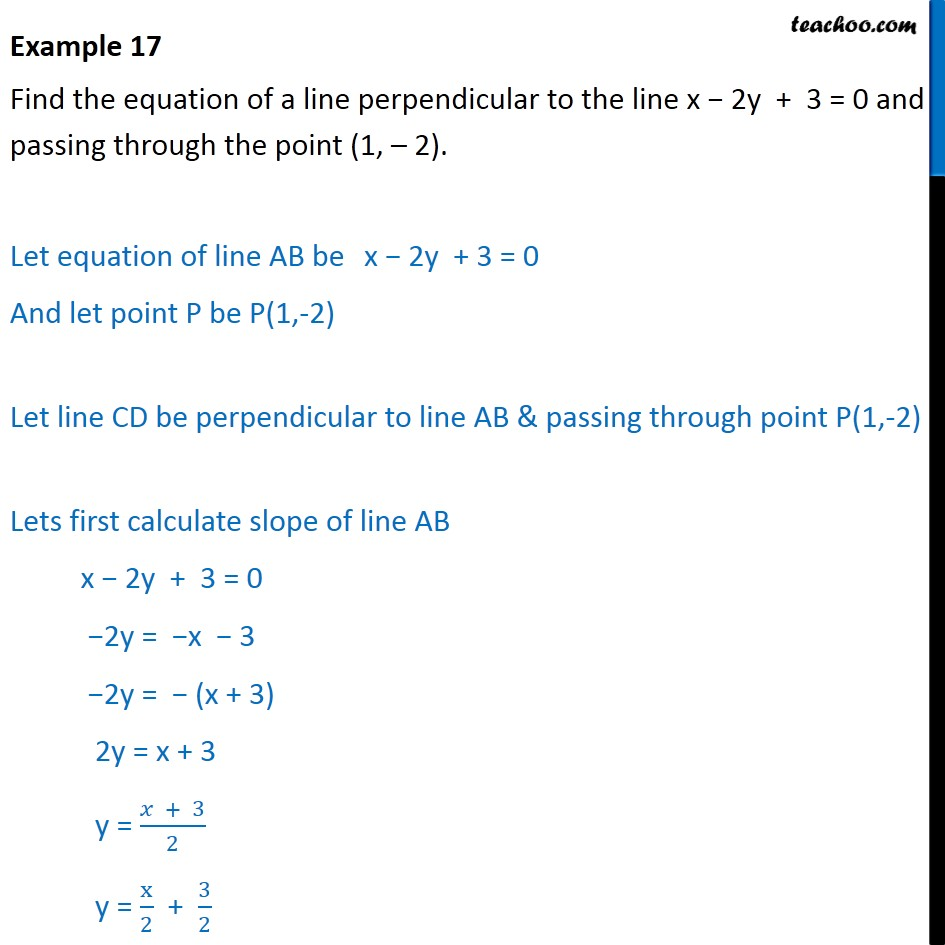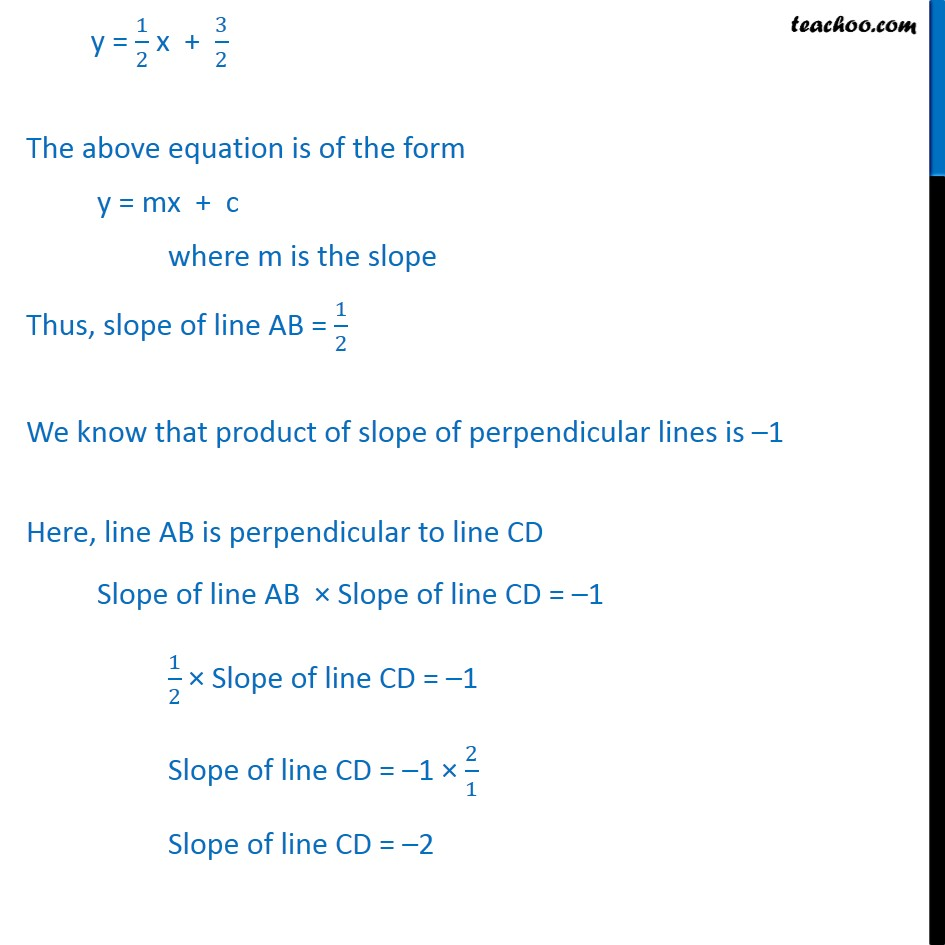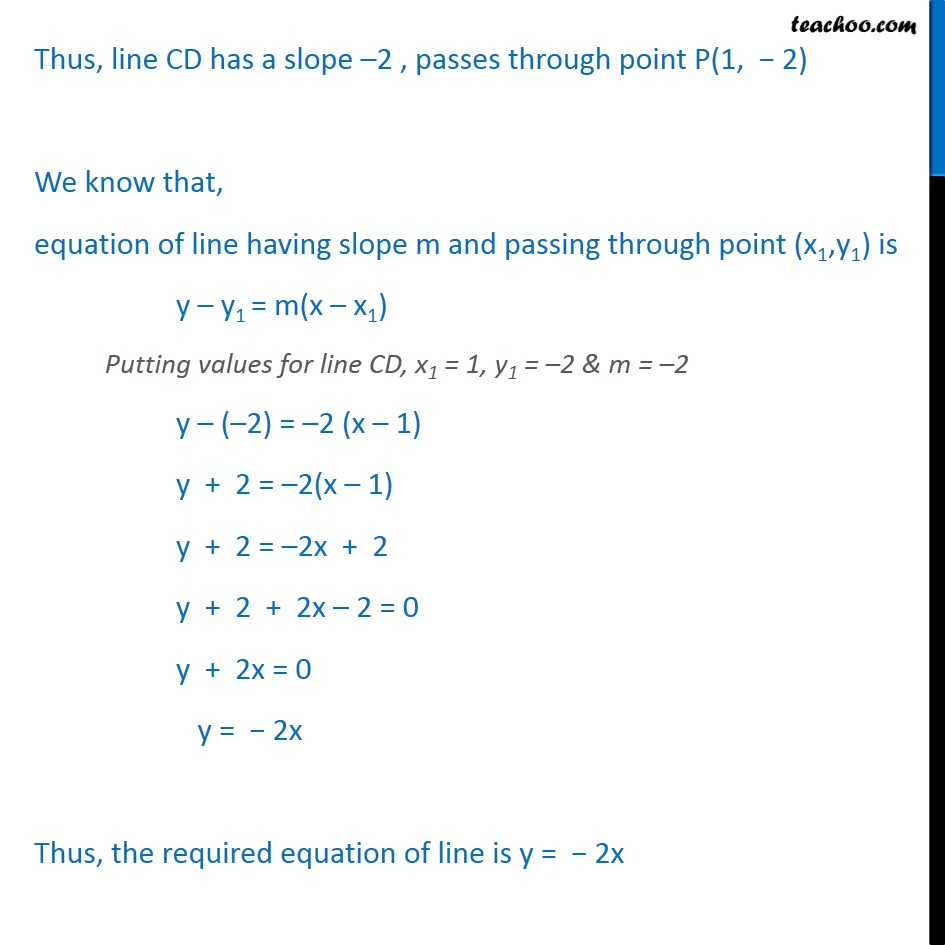Examples

Chapter 10 Class 11 Straight Lines (Term 1)
Serial order wise641 students have Teachoo Black. What are you waiting for?

### Transcript

Example 17 Find the equation of a line perpendicular to the line x 2y + 3 = 0 and passing through the point (1, 2). Let equation of line AB be x 2y + 3 = 0 And let point P be P(1,-2) Let line CD be perpendicular to line AB & passing through point P(1,-2) Lets first calculate slope of line AB x 2y + 3 = 0 2y = x 3 2y = (x + 3) 2y = x + 3 y = ( + 3)/2 y = x/2 + 3/2 y = 1/2 x + 3/2 The above equation is of the form y = mx + c where m is the slope Thus, slope of line AB = 1/2 We know that product of slope of perpendicular lines is 1 Here, line AB is perpendicular to line CD Slope of line AB Slope of line CD = 1 1/2 Slope of line CD = 1 Slope of line CD = 1 2/1 Slope of line CD = 2 Thus, line CD has a slope 2 , passes through point P(1, 2) We know that, equation of line having slope m and passing through point (x1,y1) is y y1 = m(x x1) Putting values for line CD, x1 = 1, y1 = 2 & m = 2 y ( 2) = 2 (x 1) y + 2 = 2(x 1) y + 2 = 2x + 2 y + 2 + 2x 2 = 0 y + 2x = 0 y = 2x Thus, the required equation of line is y = 2x